Publicité

# Chemical Kinetics in Unit Processes

Head of Sports à Chemical Engineering Students' Association | Nirma University
23 Mar 2015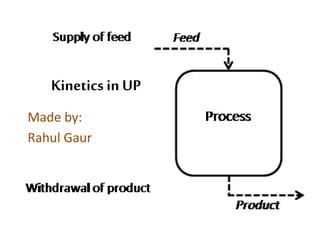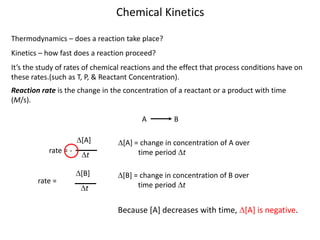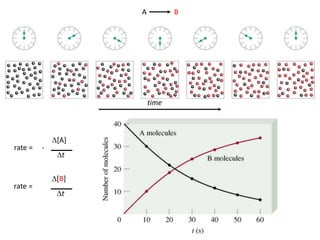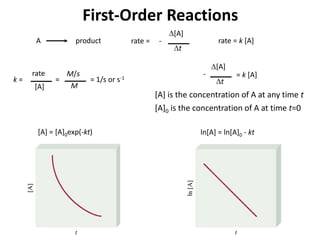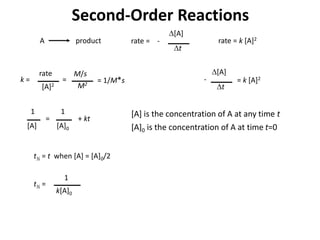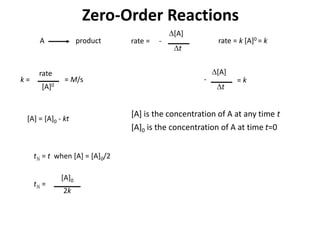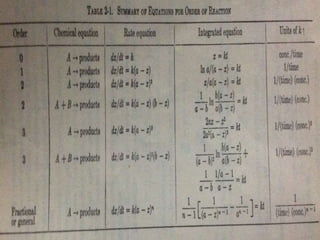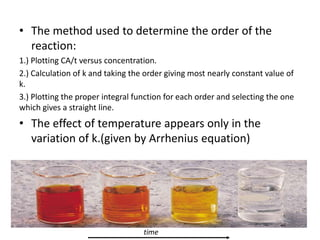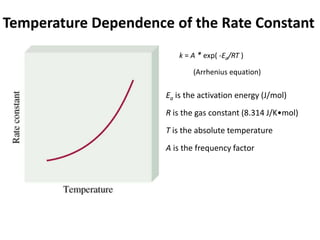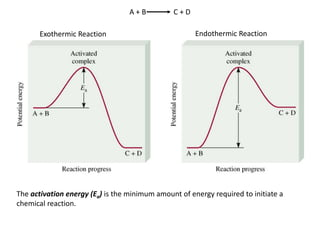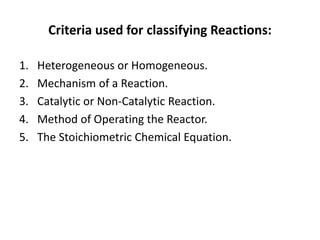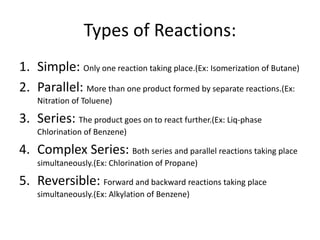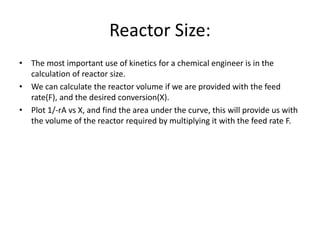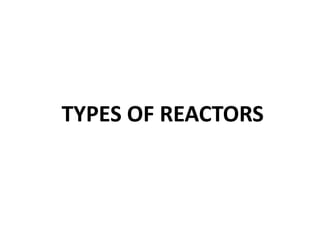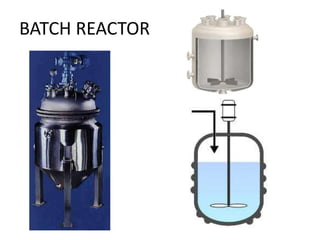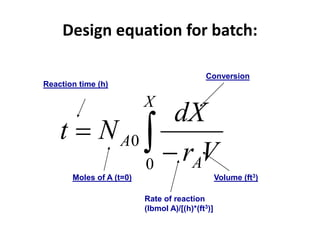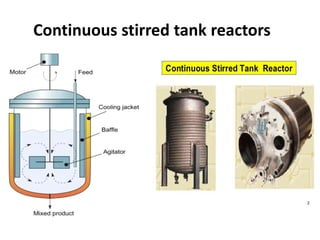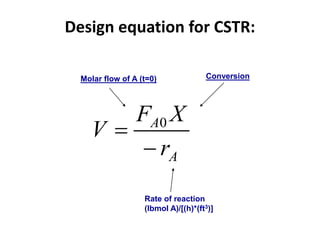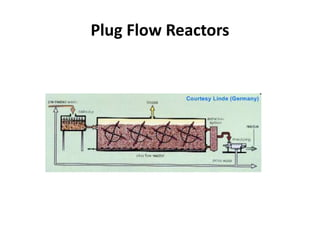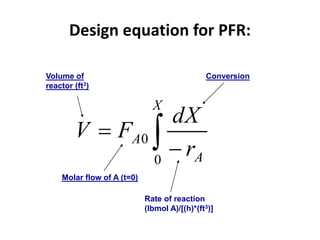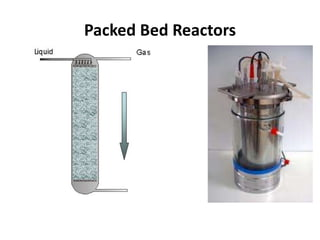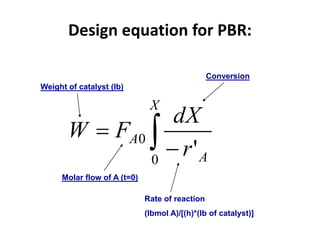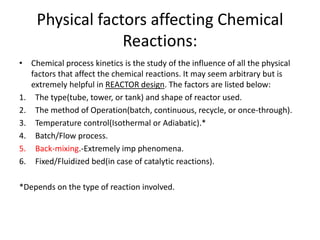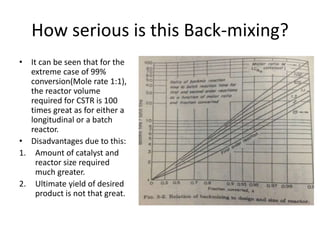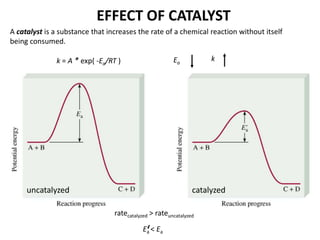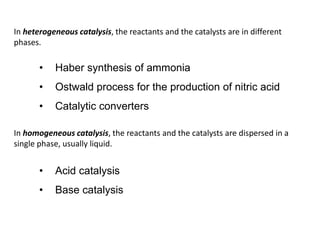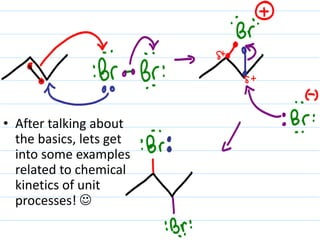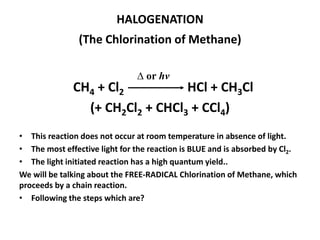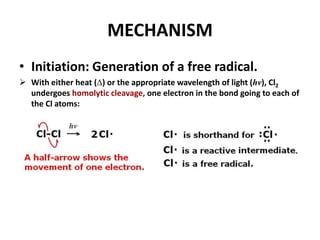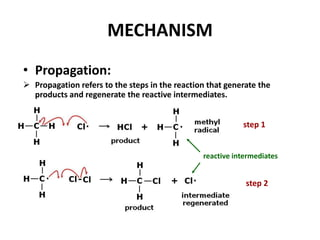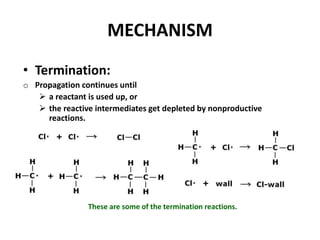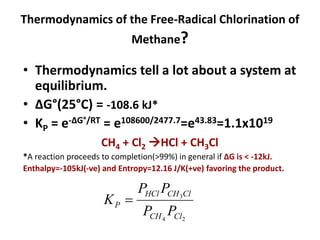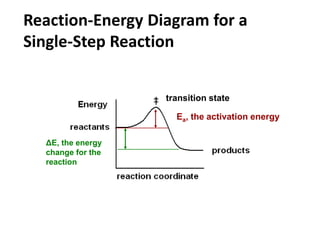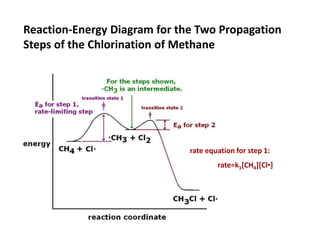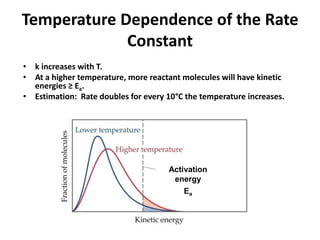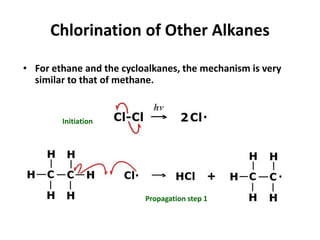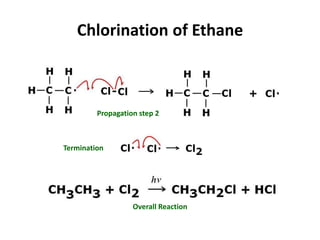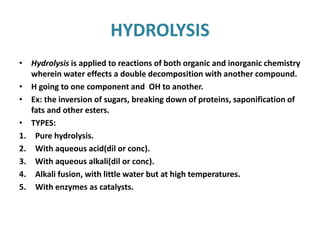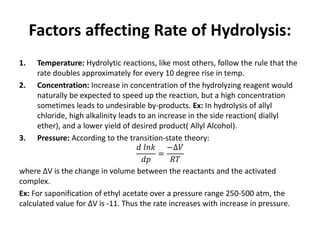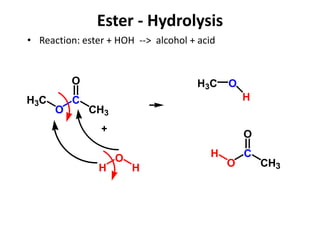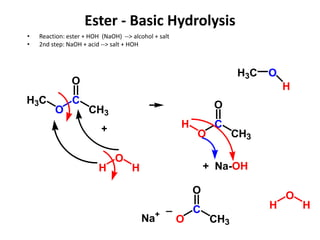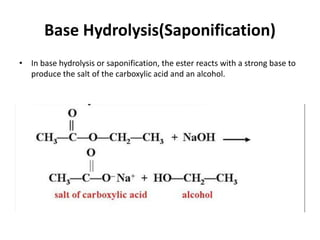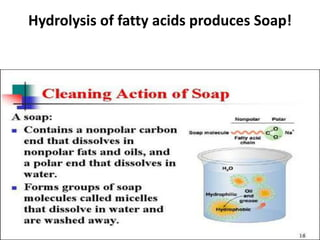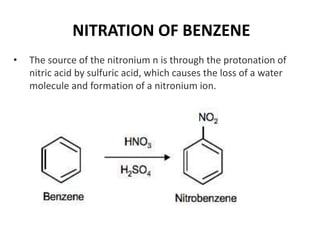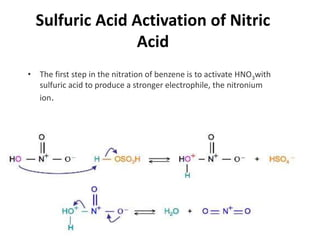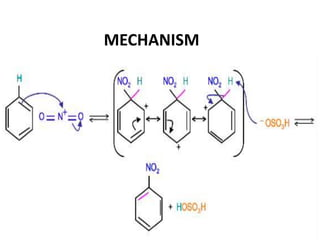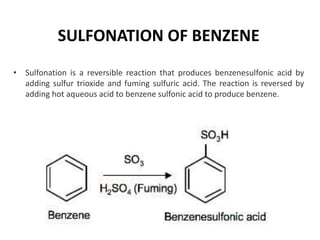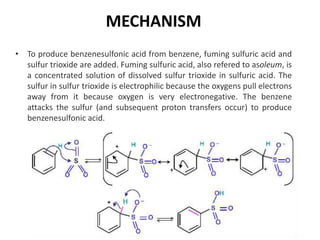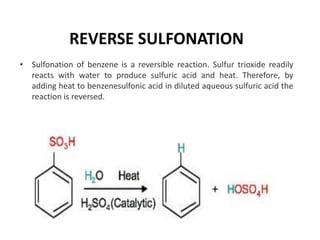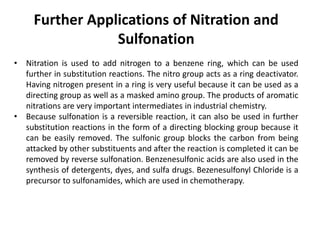1 sur 51
Publicité

### Chemical Kinetics in Unit Processes

1. Kinetics in UP Made by: Rahul Gaur
2. Chemical Kinetics Thermodynamics – does a reaction take place? Kinetics – how fast does a reaction proceed? It’s the study of rates of chemical reactions and the effect that process conditions have on these rates.(such as T, P, & Reactant Concentration). Reaction rate is the change in the concentration of a reactant or a product with time (M/s). A B rate = - D[A] Dt rate = D[B] Dt D[A] = change in concentration of A over time period Dt D[B] = change in concentration of B over time period Dt Because [A] decreases with time, D[A] is negative.
3. A B rate = - D[A] Dt rate = D[B] Dt time
4. First-Order Reactions A product rate = - D[A] Dt rate = k [A] k = rate [A] = 1/s or s-1 M/s M = D[A] Dt = k [A]- [A] is the concentration of A at any time t [A]0 is the concentration of A at time t=0 [A] = [A]0exp(-kt) ln[A] = ln[A]0 - kt
5. Second-Order Reactions A product rate = - D[A] Dt rate = k [A]2 k = rate [A]2 = 1/M*s M/s M2 = D[A] Dt = k [A]2- [A] is the concentration of A at any time t [A]0 is the concentration of A at time t=0 1 [A] = 1 [A]0 + kt t½ = t when [A] = [A]0/2 t½ = 1 k[A]0
6. Zero-Order Reactions A product rate = - D[A] Dt rate = k [A]0 = k k = rate [A]0 = M/s D[A] Dt = k- [A] is the concentration of A at any time t [A]0 is the concentration of A at time t=0 t½ = t when [A] = [A]0/2 t½ = [A]0 2k [A] = [A]0 - kt
7. • The method used to determine the order of the reaction: 1.) Plotting CA/t versus concentration. 2.) Calculation of k and taking the order giving most nearly constant value of k. 3.) Plotting the proper integral function for each order and selecting the one which gives a straight line. • The effect of temperature appears only in the variation of k.(given by Arrhenius equation) time
8. Temperature Dependence of the Rate Constant k = A * exp( -Ea/RT ) Ea is the activation energy (J/mol) R is the gas constant (8.314 J/K•mol) T is the absolute temperature A is the frequency factor (Arrhenius equation)
9. A + B C + D Exothermic Reaction Endothermic Reaction The activation energy (Ea) is the minimum amount of energy required to initiate a chemical reaction.
10. Criteria used for classifying Reactions: 1. Heterogeneous or Homogeneous. 2. Mechanism of a Reaction. 3. Catalytic or Non-Catalytic Reaction. 4. Method of Operating the Reactor. 5. The Stoichiometric Chemical Equation.
11. Types of Reactions: 1. Simple: Only one reaction taking place.(Ex: Isomerization of Butane) 2. Parallel: More than one product formed by separate reactions.(Ex: Nitration of Toluene) 3. Series: The product goes on to react further.(Ex: Liq-phase Chlorination of Benzene) 4. Complex Series: Both series and parallel reactions taking place simultaneously.(Ex: Chlorination of Propane) 5. Reversible: Forward and backward reactions taking place simultaneously.(Ex: Alkylation of Benzene)
12. Reactor Size: • The most important use of kinetics for a chemical engineer is in the calculation of reactor size. • We can calculate the reactor volume if we are provided with the feed rate(F), and the desired conversion(X). • Plot 1/-rA vs X, and find the area under the curve, this will provide us with the volume of the reactor required by multiplying it with the feed rate F.
13. TYPES OF REACTORS
14. BATCH REACTOR
15. Design equation for batch:    X A A Vr dX Nt 0 0 Conversion Volume (ft3)Moles of A (t=0) Rate of reaction (lbmol A)/[(h)*(ft3)] Reaction time (h)
16. Continuous stirred tank reactors
17. Design equation for CSTR: A A r XF V   0 ConversionMolar flow of A (t=0) Rate of reaction (lbmol A)/[(h)*(ft3)]
18. Plug Flow Reactors
19. Design equation for PFR:    X A A r dX FV 0 0 Conversion Molar flow of A (t=0) Rate of reaction (lbmol A)/[(h)*(ft3)] Volume of reactor (ft3)
20. Packed Bed Reactors
21. Design equation for PBR:    X A A r dX FW 0 0 ' Conversion Molar flow of A (t=0) Rate of reaction (lbmol A)/[(h)*(lb of catalyst)] Weight of catalyst (lb)
22. Physical factors affecting Chemical Reactions: • Chemical process kinetics is the study of the influence of all the physical factors that affect the chemical reactions. It may seem arbitrary but is extremely helpful in REACTOR design. The factors are listed below: 1. The type(tube, tower, or tank) and shape of reactor used. 2. The method of Operation(batch, continuous, recycle, or once-through). 3. Temperature control(Isothermal or Adiabatic).* 4. Batch/Flow process. 5. Back-mixing.-Extremely imp phenomena. 6. Fixed/Fluidized bed(in case of catalytic reactions). *Depends on the type of reaction involved.
23. How serious is this Back-mixing? • It can be seen that for the extreme case of 99% conversion(Mole rate 1:1), the reactor volume required for CSTR is 100 times great as for either a longitudinal or a batch reactor. • Disadvantages due to this: 1. Amount of catalyst and reactor size required much greater. 2. Ultimate yield of desired product is not that great.
24. A catalyst is a substance that increases the rate of a chemical reaction without itself being consumed. k = A * exp( -Ea/RT ) Ea k uncatalyzed catalyzed ratecatalyzed > rateuncatalyzed Ea < Ea‘ EFFECT OF CATALYST
25. In heterogeneous catalysis, the reactants and the catalysts are in different phases. In homogeneous catalysis, the reactants and the catalysts are dispersed in a single phase, usually liquid. • Haber synthesis of ammonia • Ostwald process for the production of nitric acid • Catalytic converters • Acid catalysis • Base catalysis
26. • After talking about the basics, lets get into some examples related to chemical kinetics of unit processes! 
27. HALOGENATION (The Chlorination of Methane) CH4 + Cl2 HCl + CH3Cl (+ CH2Cl2 + CHCl3 + CCl4) • This reaction does not occur at room temperature in absence of light. • The most effective light for the reaction is BLUE and is absorbed by Cl2. • The light initiated reaction has a high quantum yield.. We will be talking about the FREE-RADICAL Chlorination of Methane, which proceeds by a chain reaction. • Following the steps which are? ∆ or hν
28. MECHANISM • Initiation: Generation of a free radical.  With either heat (∆) or the appropriate wavelength of light (hν), Cl2 undergoes homolytic cleavage, one electron in the bond going to each of the Cl atoms:
29. MECHANISM • Propagation:  Propagation refers to the steps in the reaction that generate the products and regenerate the reactive intermediates. step 1 step 2 reactive intermediates
30. MECHANISM • Termination: o Propagation continues until  a reactant is used up, or  the reactive intermediates get depleted by nonproductive reactions. These are some of the termination reactions.
31. Thermodynamics of the Free-Radical Chlorination of Methane? • Thermodynamics tell a lot about a system at equilibrium. • ∆G°(25°C) = -108.6 kJ* • KP = e-∆G°/RT = e108600/2477.7=e43.83=1.1x1019 CH4 + Cl2 HCl + CH3Cl *A reaction proceeds to completion(>99%) in general if ∆G is < -12kJ. Enthalpy=-105kJ(-ve) and Entropy=12.16 J/K(+ve) favoring the product. 24 3 ClCH ClCHHCl P PP PP K 
32. Ea, the activation energy ΔE, the energy change for the reaction Reaction-Energy Diagram for a Single-Step Reaction transition state
33. Reaction-Energy Diagram for the Two Propagation Steps of the Chlorination of Methane rate equation for step 1: rate=k1[CH4][Cl▪]
34. Temperature Dependence of the Rate Constant • k increases with T. • At a higher temperature, more reactant molecules will have kinetic energies ≥ Ea. • Estimation: Rate doubles for every 10°C the temperature increases. Activation energy Ea
35. Chlorination of Other Alkanes • For ethane and the cycloalkanes, the mechanism is very similar to that of methane. Initiation Propagation step 1
36. Chlorination of Ethane Termination Propagation step 2 Overall Reaction
37. HYDROLYSIS • Hydrolysis is applied to reactions of both organic and inorganic chemistry wherein water effects a double decomposition with another compound. • H going to one component and OH to another. • Ex: the inversion of sugars, breaking down of proteins, saponification of fats and other esters. • TYPES: 1. Pure hydrolysis. 2. With aqueous acid(dil or conc). 3. With aqueous alkali(dil or conc). 4. Alkali fusion, with little water but at high temperatures. 5. With enzymes as catalysts.
38. Factors affecting Rate of Hydrolysis: 1. Temperature: Hydrolytic reactions, like most others, follow the rule that the rate doubles approximately for every 10 degree rise in temp. 2. Concentration: Increase in concentration of the hydrolyzing reagent would naturally be expected to speed up the reaction, but a high concentration sometimes leads to undesirable by-products. Ex: In hydrolysis of allyl chloride, high alkalinity leads to an increase in the side reaction( diallyl ether), and a lower yield of desired product( Allyl Alcohol). 3. Pressure: According to the transition-state theory: 𝑑 𝑙𝑛𝑘 𝑑𝑝 = −∆𝑉 𝑅𝑇 where ΔV is the change in volume between the reactants and the activated complex. Ex: For saponification of ethyl acetate over a pressure range 250-500 atm, the calculated value for ΔV is -11. Thus the rate increases with increase in pressure.
39. Ester - Hydrolysis • Reaction: ester + HOH --> alcohol + acid H O H C CH3 O O H3C + H3C O H C CH3 O O H
40. Ester - Basic Hydrolysis • Reaction: ester + HOH (NaOH) --> alcohol + salt • 2nd step: NaOH + acid --> salt + HOH H O H C CH3 O O H3C + H3C O H C CH3 O O H + Na-OH H O HC CH3 O O _ Na+
41. Base Hydrolysis(Saponification) • In base hydrolysis or saponification, the ester reacts with a strong base to produce the salt of the carboxylic acid and an alcohol.
42. Hydrolysis of fatty acids produces Soap!
43. NITRATION OF BENZENE • The source of the nitronium n is through the protonation of nitric acid by sulfuric acid, which causes the loss of a water molecule and formation of a nitronium ion.
44. Sulfuric Acid Activation of Nitric Acid • The first step in the nitration of benzene is to activate HNO3with sulfuric acid to produce a stronger electrophile, the nitronium ion.
45. MECHANISM
46. SULFONATION OF BENZENE • Sulfonation is a reversible reaction that produces benzenesulfonic acid by adding sulfur trioxide and fuming sulfuric acid. The reaction is reversed by adding hot aqueous acid to benzene sulfonic acid to produce benzene.
47. MECHANISM • To produce benzenesulfonic acid from benzene, fuming sulfuric acid and sulfur trioxide are added. Fuming sulfuric acid, also refered to asoleum, is a concentrated solution of dissolved sulfur trioxide in sulfuric acid. The sulfur in sulfur trioxide is electrophilic because the oxygens pull electrons away from it because oxygen is very electronegative. The benzene attacks the sulfur (and subsequent proton transfers occur) to produce benzenesulfonic acid.
48. REVERSE SULFONATION • Sulfonation of benzene is a reversible reaction. Sulfur trioxide readily reacts with water to produce sulfuric acid and heat. Therefore, by adding heat to benzenesulfonic acid in diluted aqueous sulfuric acid the reaction is reversed.
49. Further Applications of Nitration and Sulfonation • Nitration is used to add nitrogen to a benzene ring, which can be used further in substitution reactions. The nitro group acts as a ring deactivator. Having nitrogen present in a ring is very useful because it can be used as a directing group as well as a masked amino group. The products of aromatic nitrations are very important intermediates in industrial chemistry. • Because sulfonation is a reversible reaction, it can also be used in further substitution reactions in the form of a directing blocking group because it can be easily removed. The sulfonic group blocks the carbon from being attacked by other substituents and after the reaction is completed it can be removed by reverse sulfonation. Benzenesulfonic acids are also used in the synthesis of detergents, dyes, and sulfa drugs. Bezenesulfonyl Chloride is a precursor to sulfonamides, which are used in chemotherapy.

1. 28
2. 30
3. 31
4. 32
5. 33
6. 34
7. 35
8. 36
9. 37
Publicité